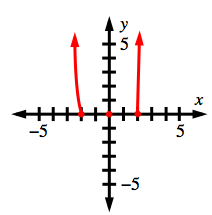### Home > CCA2 > Chapter Ch10 > Lesson 10.3.1 > Problem10-151

10-151.

Sketch a graph of $y = x^3(x - 2)(x + 2)^2$

What are the $x$- and $y$-intercepts?
How does the function behave at each $x$-intercept?

What is the end behavior of the function?